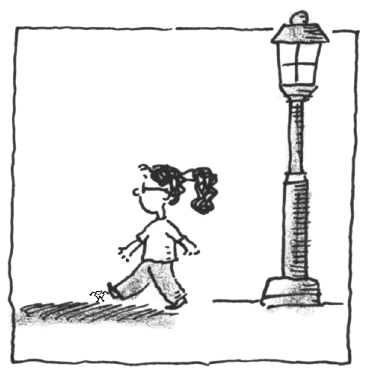### Home > APCALC > Chapter 11 > Lesson 11.1.2 > Problem11-26

11-26.

Multiple Choice: A $4$-foot tall child walks away from a $12$‑foot tall street light at a rate of $2$ ft/sec. At what rate is the length of her shadow increasing when she is $6$ feet away from the light?

1. $1$ ft/sec

1. $2$ ft/sec

1. $3$ ft/sec

1. $4$ ft/sec

1. $6$ ft/secSketch the situation. Your sketch should have similar right triangles.

If the child's distance from the light is $d$ and their shadow length is $s$, then:

$\frac{12}{s+d}=\frac{4}{s}$

The equation can be rewritten as $2s = d$.
Now differentiate.

$2\frac{ds}{dt}=\frac{dd}{dt}$

Now use the information given in the problem to solve for $ds/dt$.